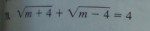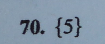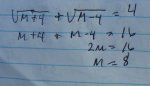what is happening here?

allegansveritatem

Full Member
This seems to be a very simple equation...but somehow the solution is eluding me. I have tried again and again and can't get it to come out right. lIt is easy to see what the answer should be without working it out. But when I try to work it out, Mr Right doesn't show up.

Equation:What I keep getting:Dr.Peterson

Elite Member
This seems to be a very simple equation...but somehow the solution is eluding me. I have tried again and again and can't get it to come out right. lIt is easy to see what the answer should be without working it out. But when I try to work it out, Mr Right doesn't show up.

Equation:
View attachment 10826

View attachment 10827

What I keep getting:

View attachment 10828
When you square both sides, you have to square each entire side -- not just each term on each side.

The square of $$\displaystyle \sqrt{m+4}+\sqrt{m-4}$$ is not $$\displaystyle \sqrt{m+4}^2+\sqrt{m-4}^2$$, but $$\displaystyle (\sqrt{m+4}+\sqrt{m-4})^2$$. Do you see the difference?

Doing that will not simplify the equation. What you have to do is to isolate one radical (move the other to the right side), and then square each side.

Your textbook should have examples of this.

HallsofIvy

Elite Member
$$\displaystyle (a+ b)^2= a^2+ 2ab+ b^2$$, not $$\displaystyle a^2+ b^2$$.

$$\displaystyle (\sqrt{m+4}+ \sqrt{m-4})^2=$$
$$\displaystyle (\sqrt{m+4})^2+ 2\sqrt{m+4}\sqrt{m-4}+ (\sqrt{m-4})^2$$
$$\displaystyle = m+ 4+ 2\sqrt{(m+4)(m-4)}+ m- 4= 2m+ 2\sqrt{m^2- 16}$$.

So the equation, after squaring both sides, is $$\displaystyle 2m+ 2\sqrt{m^2- 16}= 16$$. Rewrite this as $$\displaystyle \sqrt{m^2- 16}= 8- m$$ and square both sides again to get an equation for m.

Caution: While any solution to the original equation must satisfy the new equation, squaring both sides of an equation can introduce "spurious solutions"- solutions to the new equation that do not satisfy the original equation. Be sure to check any solutions to your final equation in the original equation!

Last edited:

tkhunny

Moderator
Staff member
This seems to be a very simple equation...but somehow the solution is eluding me. I have tried again and again and can't get it to come out right. lIt is easy to see what the answer should be without working it out. But when I try to work it out, Mr Right doesn't show up.

Equation:
View attachment 10826

View attachment 10827

What I keep getting:

View attachment 10828
Please learn to experiment with simpler examples. Maybe...

$$\displaystyle 4 + 3 = 7$$

$$\displaystyle 16 + 9 \ne 49$$ -- Something went wrong.

Jomo

Elite Member
Please learn to experiment with simpler examples. Maybe...

$$\displaystyle 4 + 3 = 7$$

$$\displaystyle 16 + 9 \ne 49$$ -- Something went wrong.
I 2nd that technique.

I turd it!

allegansveritatem

Full Member
When you square both sides, you have to square each entire side -- not just each term on each side.

The square of $$\displaystyle \sqrt{m+4}+\sqrt{m-4}$$ is not $$\displaystyle \sqrt{m+4}^2+\sqrt{m-4}^2$$, but $$\displaystyle (\sqrt{m+4}+\sqrt{m-4})^2$$. Do you see the difference?

Doing that will not simplify the equation. What you have to do is to isolate one radical (move the other to the right side), and then square each side.

Your textbook should have examples of this.
I will look at this more closely in the morning but I see what is being said..and also, I know that squaring this type of problem with all the radicals on one side is not a means to an easy life. In fact I did try to move one of the radical terms to the right but somehow things seemed to end up exactly the same. But I will go at this again tomorrow and keep what you say in mind. Thanks for pointing out the problem. I think I did it this way in the hope of avoiding a messy FOIL.

allegansveritatem

Full Member
$$\displaystyle (a+ b)^2= a^2+ 2ab+ b^2$$, not $$\displaystyle a^2+ b^2$$.

$$\displaystyle (\sqrt{m+4}+ \sqrt{m-4})^2=$$
$$\displaystyle (\sqrt{m+4})^2+ 2\sqrt{m+4}\sqrt{m-4}+ (\sqrt{m-4})^2$$
$$\displaystyle = m+ 4+ 2\sqrt{(m+4)(m-4)}+ m- 4= 2m+ 2\sqrt{m^2- 16}$$.

So the equation, after squaring both sides, is $$\displaystyle 2m+ 2\sqrt{m^2- 16}= 16$$. Rewrite this as $$\displaystyle \sqrt{m^2- 16}= 8- m$$ and square both sides again to get an equation for m.

Caution: While any solution to the original equation must satisfy the new equation, squaring both sides of an equation can introduce "spurious solutions"- solutions to the new equation that do not satisfy the original equation. Be sure to check any solutions to your final equation in the original equation!
Thanks for working through it...I will get back to this in the morning when my brain comes back from the dead. I do know about the fdalse solution pitfall, and have actually fallen into it more than once.

allegansveritatem

Full Member
Please learn to experiment with simpler examples. Maybe...

$$\displaystyle 4 + 3 = 7$$

$$\displaystyle 16 + 9 \ne 49$$ -- Something went wrong.
I know this technique and I assure you I use it all the time. I use 2 and 3 as my dummies of choice

Otis

Senior Member
… I think I did it this way in the hope of avoiding a messy FOIL.
There are no shortcuts to any place worth going. :cool:

Denis

Senior Member
Kinda sloppy, but correct...you get a pink star for your forehead :razz:

JeffM

Elite Member
This is not correct.

$$\displaystyle a + b > c \text { DOES NOT GENERALLY ENTAIL } a > b + c.$$

Furthermore, by definition $$\displaystyle \sqrt{x} \in \mathbb R \implies 0 \le \sqrt{x}.$$

So, $$\displaystyle - 3 = \sqrt{m + 4}$$ is impossible.

EDIT: You can sometimes get a correct answer through an incorrect process, but do not count on it.

Last edited:

Jomo

Elite Member
No, this is not correct. First when you moved sqrt(m+4) to the other side you failed to change the sign. 2nd mistake is that you got a solution to sqrt(m+4) = -3 !! The sqrt of nothing is ever negative. Go back and fix your mistakes.

Jomo

Elite Member
Kinda sloppy, but correct...you get a pink star for your forehead :razz:
Correct??? Go to the corner for a few hours and review your algebra as you are clearly a bit rusty.

Denis

Senior Member
Problem posted: sqrt(M + 4) + sqrt(M - 4) = 4

All I did was substitute his solution M=5 to get sqrt(9)+sqrt(1) = 3+1 = 4

Why is M=5 wrong?

Dr.Peterson

Elite Member
Problem posted: sqrt(M + 4) + sqrt(M - 4) = 4

All I did was substitute his solution M=5 to get sqrt(9)+sqrt(1) = 3+1 = 4

Why is M=5 wrong?
Five is right. But along the way, two mistakes are made that cancel one another out. Getting the right answer in an invalid way doesn't count as correct.

allegansveritatem

Full Member
This is not correct.

$$\displaystyle a + b > c \text { DOES NOT GENERALLY ENTAIL } a > b + c.$$

Furthermore, by definition $$\displaystyle \sqrt{x} \in \mathbb R \implies 0 \le \sqrt{x}.$$

So, $$\displaystyle - 3 = \sqrt{m + 4}$$ is impossible.

EDIT: You can sometimes get a correct answer through an incorrect process, but do not count on it.
I'm sorry but this needs to be unpacked a little so a novice like me can make it out.

allegansveritatem

Full Member
No, this is not correct. First when you moved sqrt(m+4) to the other side you failed to change the sign. 2nd mistake is that you got a solution to sqrt(m+4) = -3 !! The sqrt of nothing is ever negative. Go back and fix your mistakes.
Yes, I see that now. I mean, I see that I forgot to change the sign when I switched the radical over to the right. That is a huge mistake right there and yet...the answer came out right. So....I must either have done something else that cancelled that error or maybe the nature of this type of problem is such that flukes of this sort are, if not likely, yet still possible. Anyway, tomorrow I will have another lunge at this beast, cuz it ain't dead yet!